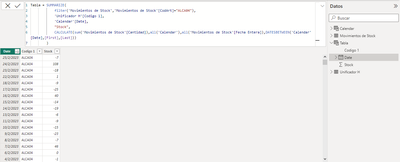cancel
Showing results for
Did you mean:New Member

## Counting every time any product´s stock hits 0

Hi everyone!

I have a database of every movement of every product our company owns. This means that if I make a cumulative sum meassure, I get the stock at every point in time, of any article. What I want now is a meassure that calculates how many times a product hits 0 in any given period of time. I want, however, this meassure to be completely adaptable to any filter and context it is applied to. If I show it in a graph where I show the frequency of this event, but for a Brand with more than one product, I want it to show how many times each article hits 0. I also need this to work in a simple card, where if I select a time interval and a brand or group of products, it needs to tell me how, for every product and every date, how many times the stock was 0.Here is my current progress, where I try to achieve this via a summarize table, but as you can see, it won´t do a cummulative sum and so I can´t proceed with the measure.

I don´t know if this is the way, but I will accept any solution to this problem.

Thanks!

1 ACCEPTED SOLUTIONCommunity Support

Hi @bacosta99 ,

I think you can try EARLIER() function to calculate stock value.

``````Tabla =
SUMMARIZE (
FILTER ( 'Movimientos de Stock', 'Movimientos de Stock'[CodArt] = 'ALCA04' ),
'Calendar'[Date],
"Stock",
CALCULATE (
SUM ( 'Movimientos de Stock'[Cantidad] ),
FILTER (
'Movimientos de Stock',
'Movimientos de Stock'[CodArt] = EARLIER ( [CodArt] )
&& 'Movimientos de Stock'[Date] <= EARLIER ( [Date] )
)
)
)``````

Best Regards,
Rico Zhou

If this post helps, then please consider Accept it as the solution to help the other members find it more quickly.Community Support

Hi @bacosta99 ,

I think you can try EARLIER() function to calculate stock value.

``````Tabla =
SUMMARIZE (
FILTER ( 'Movimientos de Stock', 'Movimientos de Stock'[CodArt] = 'ALCA04' ),
'Calendar'[Date],
"Stock",
CALCULATE (
SUM ( 'Movimientos de Stock'[Cantidad] ),
FILTER (
'Movimientos de Stock',
'Movimientos de Stock'[CodArt] = EARLIER ( [CodArt] )
&& 'Movimientos de Stock'[Date] <= EARLIER ( [Date] )
)
)
)``````

Best Regards,
Rico Zhou

If this post helps, then please consider Accept it as the solution to help the other members find it more quickly.